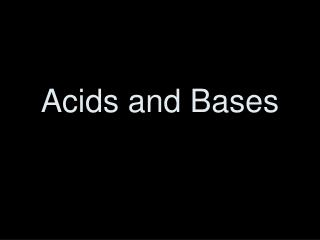DownloadDownload PresentationAcids and Bases

# Acids and Bases

Download Presentation## Acids and Bases

- - - - - - - - - - - - - - - - - - - - - - - - - - - E N D - - - - - - - - - - - - - - - - - - - - - - - - - - -
##### Presentation Transcript

1. Acids and Bases

2. Drill – 4/23/08 • Write everything you know about acids and bases!

3. Drill – 4/30/08 • Write the equation for the neutralization reaction between carbonic acid and calcium hydroxide.

4. Properties of Acids (in aq solution) • Sour taste • Low pH • Turn litmus paper red • Conduct electrical current

5. Properties of Bases (in aq solution) • Bitter taste • Slippery • High pH • Turn litmus paper blue • Conduct electrical current

6. Arrhenius Definition Acid = substance that releases H+ ions in aq solution HCl  H+ + Cl- Base = substance that releases OH-ions in aq solution NaOH  Na+ + OH-

7. Bronsted-Lowry Definition As more and more substances were determined to have acidic or basic properties, even some without obvious H+ or OH-, a new definition was needed. • Acid = proton donor • Base = proton acceptor

8. Bronsted-Lowry Definition • Acid = proton donor H2O + HCl  H3O+1 + Cl-1

9. Bronsted-Lowry Definition • Base = proton acceptor NH3 + H2O  NH4+ + OH–

10. Acid-Base Reactions • Known as a neutralization reaction. Acids and bases will react with each other to form water and salt. HCl + NaOH  H2O + NaCl

11. Day 2

12. Acid Naming Overview Binary Start with hydro- Element End with –ic acid Tertiary “I ate something, and now I am sick” If anion ends in –ate then the acid ends in –ic If the anion ends in –ite then the acid ends in -ous

13. Acids & Bases Review • Acid • substance that releases H+ ions in aq solution • Proton donor • Electron pair acceptor • Base • substance that releases OH-ions in aq solution • Proton acceptor • Electron pair donator

14. Acid Strength A stronger acid will transfer MORE protons (H+) than a weak acid. It will create more hydronium ions (H30+) in water. Hydrochloric acid HCl + H20  H30+ + Cl- Acetic Acid CH3COOH + H20 H30+ + CH3COO- More dissociation! Less dissociation!

15. How do we compare the strength of an acid or a base? We measure the amount of hydronium ions they create (acids) or the amount of hydroxide ions they create (bases)….using concentration.

16. What is concentration? • Using molarity, it is a measure of moles of solute in liters of solution. • Concentration is measured in WATER!

17. H2O is both an acid and a base Water is simultaneously donating and accepting protons!

18. What are the ion concentrations in water? • The concentrations of H3O+ and OH- in pure water are each 1.0x10-7 mol/L at 25ºC. • Putting a symbol in brackets is used to signify the concentration. • [H30+] = 1.0x10-7 M • [OH-] = 1.0x10-7 M

19. Ionization Constant of water, KW • KW = [H30+] [OH-] • KW = (1.0x10-7)(1.0x10-7) = 1.0x10-14

20. With an increase in [H30+], some of the H30+ ions will react with the OH- ions, reducing [OH-] . [H30+][OH-] will still = 1.0x10-14 • Acids increase the [H30+] Whenever [H30+] is greater than [OH-], the solution is acidic. • Bases increase the [OH-] Whenever [OH-] is greater than [H30+], the solution is basic.

21. Concentration values tend to be small…so we use a more convenient scale. pH!

22. pH + pOH = 14 • pH – A measure of the acidity of a solution. It is the negative logarithm of the hydronium ion [H30+] concentration. pH = -log [H30+]

23. pH + pOH = 14 • pOH – A measure of how basic a solution is. It is the negative logarithm of the hydroxide [OH-] concentration. pOH = -log [OH-]

24. The logarithm base 10 of a number x is the power to which 10 must be raised in order to equal x. • Log 10 x = ? Log 100 = ? • 10? = x 10 ? = 100

25. Remember, our concentrations are SMALL. So we are going to be dealing with decimals… • Log 10 x = ? Log .001 = ? • 10? = x 10 ? = .001

26. [H+] = 1.00 x 10-3 = 0.001 pH = -log [H+] pH = -log (1.00 x 10-3) pH = 3 To determine pH from a concentration where 1 is the only digit, write the concentration in scientific notation. The exponent will be the pH.

27. For a base, you do the same thing except remember that you are solving for pOH first…